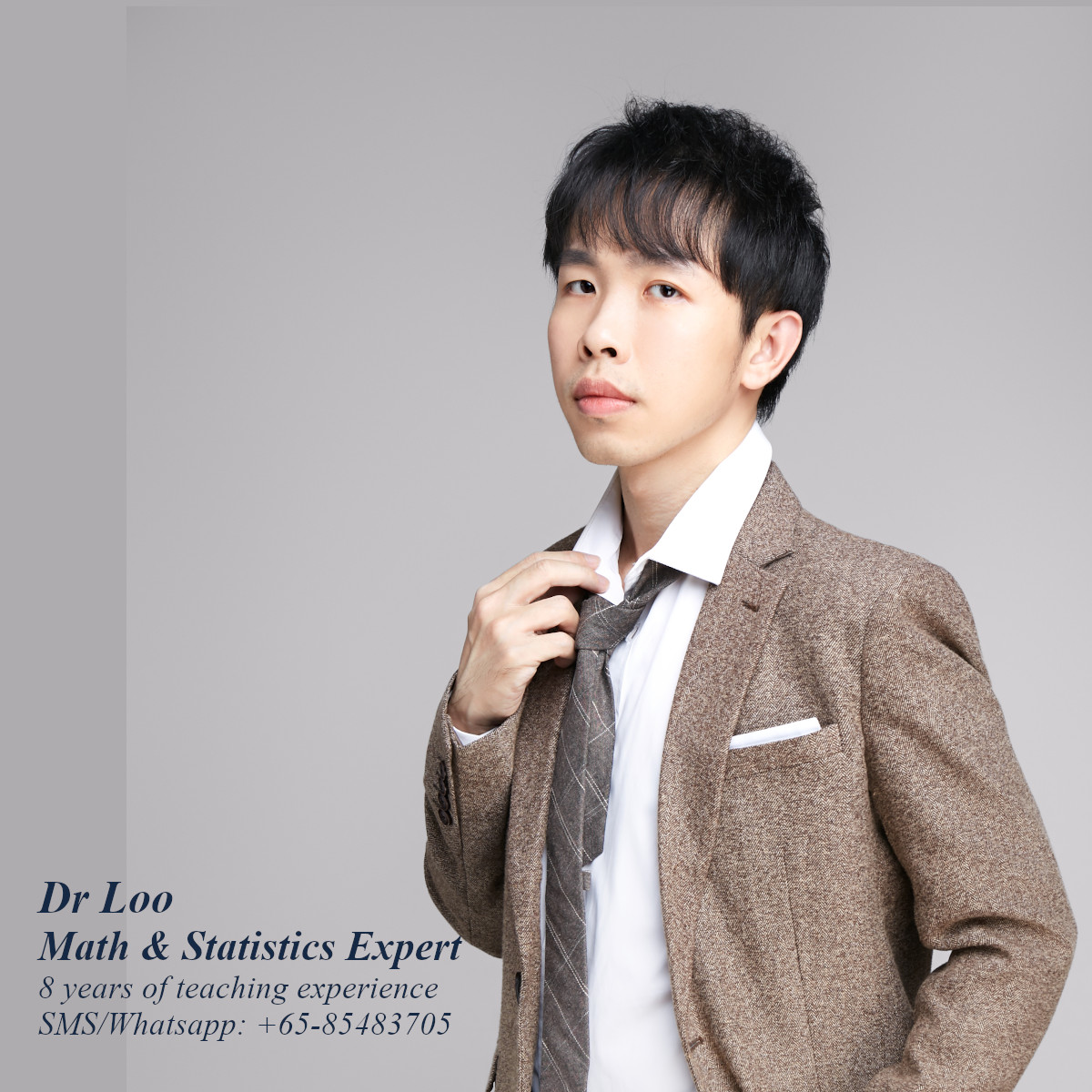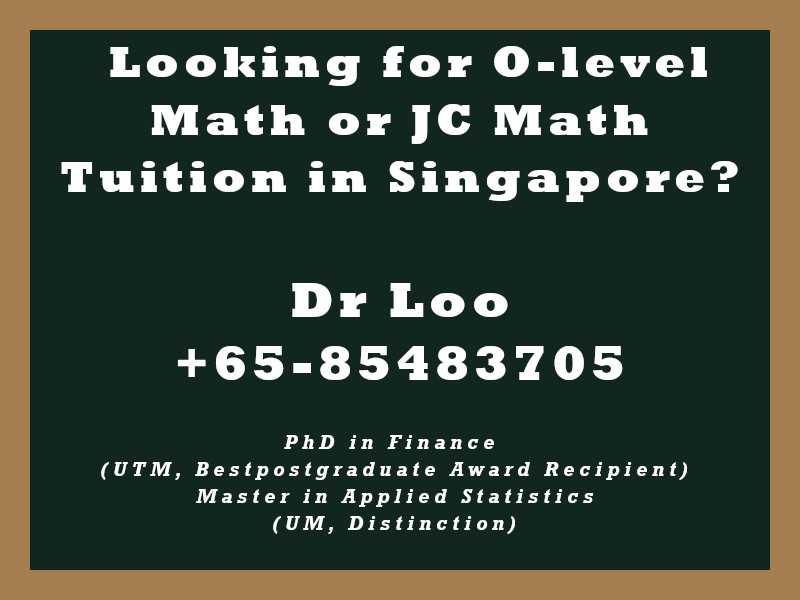+65-85483705

# Inverse Function

This topic is introduced when a student is learning about a function. They will learn what is the definition of the function. In addition to that, there are certain conditions which a function has its inverse. This will be covered in JC H2 Mathematics class.

Question

For each of the functions, obtain its inverse function.

1. g(x) = 2/x
2. g(x) = x + 1
3. g(x) = 3/(x-1)

1. g-1(x) = 2/x
2. g-1(x) = x-1
3. g-1(x) = (3/x) + 1

Useful Notes:

• In order to obtain the inverse function, first one need to set the function x as y then make y become the subject of the formula.
• Function f is said to be the inverse of function g if the inverse of function f is also equal to function g and vice versa.
• For verification purpose, one can check if ff-1(x) or f-1f(x) will return to x.

## About the Singapore Math Tutor - Dr Loo

I am a PhD holder with 9 years of teaching experience on teaching quantitative subjects at secondary school and university levels. My expertise are mathematics, statistics, econometrics, finance and machine learning.

Currently I do provide consultation and tuition for math tuition in Singapore. If you are looking for secondary math tutor in Singapore, JC H2 math tutor or statistics tutor in Singapore, please feel free to contact me at +65-85483705 (SMS/Whatsapp).

Among the Singapore math tuition that I provide, this includes Singapore secondary math tuition (E-math tuition & A-math tuition) and also JC Math Tuition (H1 Math Tuition & H2 Math Tuition). On the other hand, my statistics tuition in Singapore mostly focus on pre-university level until postgraduate level. For those who are looking for online tutoring service, the online O-level math tuition and online A-level math tuition are also available.## For those who are looking for math tution in Singapore

Need help with this topic? I do provide mathematics home tuition in Singapore for O-level math and also JC H2 math. In addition, online math tutoring is available as well. Feel free to contact me if you would like to know further.